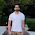## Saturday, 11 March 2017

### Hidden Markov Model: Session -2

Probabilistic inference in an HMM :-

1) Compute the probability of given observed states when the tag sequence is hidden
2) Find the most likely hidden sequence path
3) Given the observation sequence, find the parameters that will make observations most likely.

these 3 are known as Evaluation problem, Decoding problem and Learning problem.

1) Evaluation Problem-Identify all possible hidden state sequences. The sequence that generates the observed states with maximum probability is the right sequence. Lets us explain it conceptually-

w0, w1, w2.. are hidden states. t=0, t=1, t=2 are different time intervals. Transition probabilities are a11 ( transition from 1 to 1) and emission probabilities are b1( emission from state 1).

At t=0, say all 2 states w1 and w2 are present with probability .5,.5 ( not taking all hidden states). There would be 4 possible transitions.
a11, a12, a21, a22. So the next state probability would be-
hidden state being 1- .5*a11 +.5*a21
hidden state being 2- .5*a12 + .5*a22

Thus again these participation probabilities would taken for further state participation calculation. like for time t=2

hidden state being 1=  (5*a11 +.5*a21)* a11 +(.5*a12 + .5*a22) * a21
hidden state being 2 = (5*a11 +.5*a21)* a12 +(.5*a12 + .5*a22) * a22

Thus using this forward algorithm probabilities of all the hidden state sequence is generated and the sequence with maximum probability of emitting output values is considered the hidden state sequence.

This is the evaluation problem that says that given hidden state sequence has generated given output sequence with this probability.

Detailed sessions are present at- https://www.youtube.com/watch?v=E3qrns5f3Fw
Basic Info about HMM are present at- http://machinelearningstories.blogspot.in/2017/02/hidden-markov-model-session-1.html

1.1.2.